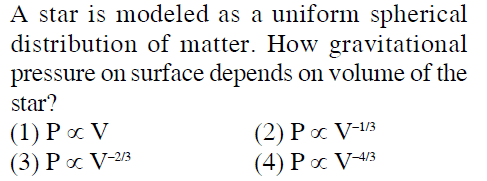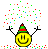# Gravitational pressure dependence on volume

## Homework Statement## The Attempt at a Solution

Consider a very thin shell of width dr at a distance r from the center . The volume of this shell is ##4 \pi r^2 ## . Mass is ## m = 4 \pi r^2 \rho## .

P is the pressure at distance r . Gravitational acceleration at distance r < R is ##g' = \frac{GMr}{R^3}##

Doing a force balance on this thin shell .

##P(4 \pi r^2) - (P+dP) (4\pi r^2) = mg'##

## - dP (4\pi r^2) = (4 \pi r^2 \rho)\frac{GMr}{R^3}##

Integrating under proper limits ,

$$P(r) = \frac{GM\rho}{2R} \left ( 1 - \frac{r^2}{R^2} \right )$$ . V is the volume of the star .

Now , at the surface P(r = R) = 0 .

Is the question wrong ? Should the question be asking about the pressure "at the center" instead of "on the surface" ?

Thanks

#### Attachments

phinds
Gold Member
2021 Award
Derive the equation for the gravitation attraction of a point on the surface in terms of radius and mass and then express radius as a function of volume. And you'll have to get rid of the mass term by expressing it in terms of volume and density (density is a constant you can ignore for the purposes of this problem)

Last edited:
Isn't gravitational pressure 0 at the surface ? Is the problem statement correct ?

phinds
Gold Member
2021 Award
Isn't gravitational pressure 0 at the surface ?
If it were, what would keep the star from just evaporating from the outside inward ? Just figure out the gravitational force pointing in and the pressure is the same force pointing out.

That gives option 3) as answer .

phinds
Gold Member
2021 Award
not what I got, but I was pretty off-the cuff w/ the math. You're probably right.

Do you get option 3) as answer ?

phinds
Gold Member
2021 Award
Do you get option 3) as answer ?
Uh ... "not what I got" was a direct response to your statement that you got #3.

If you were careful with the math, you probably got it right. Don't worry about what I got.

But I surely need to worry about how you have interpreted gravitational pressure . Sorry , but I think you have got it wrong . Gravitational pressure is 0 at the surface . It is not the same thing as gravitational force .

phinds
Gold Member
2021 Award
But I surely need to worry about how you have interpreted gravitational pressure . Sorry , but I think you have got it wrong . Gravitational pressure is 0 at the surface . It is not the same thing as gravitational force .
Well, there again you may be right. I'm no expert. I just looked up gravitational pressure and it showed a diagram with "gravitational pressure" pointing out exactly balancing "gravity" pointing in.

Also, I go under the assumption that a problem statement is not nonsense (which is not ALWAYS right but certainly ALMOST always).

Last edited:
TSny
Homework Helper
Gold Member
Doing a force balance on this thin shell .

##P(4 \pi r^2) - (P+dP) (4\pi r^2) = mg'##

##P(4 \pi r^2)## does not give the total force on a spherical surface. The net force due to pressure on a spherical surface will be zero. The pressure force on a small element of the spherical surface is opposite in direction to the force at a diametrically opposite point.

Instead, consider a small, thin cylinder of height ##dr## oriented as shown below and located a distance ##r## from the center of the star.

Now , at the surface P(r = R) = 0 .

Is the question wrong ? Should the question be asking about the pressure "at the center" instead of "on the surface" ?
Yes, I think the problem meant to ask about the pressure at the center.

There is also an ambiguity in the question. When you are determining how the pressure at the center varies with volume, does the total mass of the star remain constant as the volume varies, or does the density remain constant as the volume varies?

#### Attachments

•Vibhor
Thanks for replyingInstead, consider a small, thin cylinder of height ##dr## oriented as shown below and located a distance ##r## from the center of the star.

I get same result as in OP $$P(r) = \frac{GM\rho}{2R} \left ( 1 - \frac{r^2}{R^2} \right )$$
Yes, I think the problem meant to ask about the pressure at the center .

Ok
When you are determining how the pressure at the center varies with volume, does the total mass of the star remain constant as the volume varies, or does the density remain constant as the volume varies?

I think the later i.e density remains constant as the volume varies .

TSny
Homework Helper
Gold Member
I get same result as in OP $$P(r) = \frac{GM\rho}{2R} \left ( 1 - \frac{r^2}{R^2} \right )$$
OK, I think that's right.

I think the later i.e density remains constant as the volume varies .
If so, I don't get one of the choices.

•Vibhor
No . Looking at the options , I think it is the mass that remains constant and density varies .

If density remains constant then ## P (center) ∝ V^{\frac{2}{3}}## . But if mass remains constant then ## P (center) ∝ V^{\frac{-4}{3}}## which is an option given in the problem statement .

TSny
Homework Helper
Gold Member
No . Looking at the options , I think it is the mass that remains constant and density varies .

If density remains constant then ## P (center) ∝ V^{\frac{2}{3}}## . But if mass remains constant then ## P (center) ∝ V^{\frac{-4}{3}}## which is an option given in the problem statement .
OK. Good.

•Vibhor
Delta2
Homework Helper
Gold Member
Gravitational acceleration at distance r < R is ##g′= \frac{GMr}{R^3}##

Nope the gravitational acceleration at distance r<R is ##\frac{G\rho V_r}{r^2}## where ##V_r## is the volume of a sphere with radius r.

Nope the gravitational acceleration at distance r<R is ##\frac{G\rho V_r}{r^2}## where ##V_r## is the volume of a sphere with radius r.

##\frac{GMr}{R^3}## and##\frac{G\rho V_r}{r^2}## are equivalent•Delta2
haruspex
Homework Helper
Gold Member
I am attaching the solution provided with this problem . I can't make a head or tail of it. Can you understand how it is being done in the solution ?
No, I don't understand the reasoning there. That dW would be the work required to change the volume by dV at constant pressure P. Why that should relate the pressure at the centre of the star to the total gravitational PE I have no idea.

•Vibhor
TSny
Homework Helper
Gold Member
I am attaching the solution provided with this problem . I can't make a head or tail of it. Can you understand how it is being done in the solution ?
I don't understand this solution either. But it does appear that they are finding the pressure at the surface. PdV could represent the work done by the surface pressure on the star for a virtual change in volume dV. Then they equate this to the change in gravitational potential energy ##U## of the star. But off hand, I don't see why you can equate them.

I found some notes here http://star.pst.qub.ac.uk/~sjs/teaching/stellarevol/pdfs/Lecture 3.pdf that might be relevant.

At bottom of page 3 and top of page 4, they develop a relation which they call the "virial theorem": ##3\int_0^{V_s}{PdV} - U = 0##. But note that in deriving this result they assume the pressure is zero at the surface! It is tempting to take the derivative of this expression with respect to ##V_s## to get ##P_s =\frac{1}{3} \frac{dU}{dV_s}##. But that would imply a nonzero pressure at the surface! So, I'm not seeing what's going on here.

Hopefully someone can enlighten us.

•Vibhor
Thanks for your valuable inputs .

##P(4 \pi r^2)## does not give the total force on a spherical surface. The net force due to pressure on a spherical surface will be zero.

I think I understand what you are saying . But if what I did in OP is wrong how do I get the correct expression for pressure in the OP?

TSny
Homework Helper
Gold Member
I think I understand what you are saying . But if what I did in OP is wrong how do I get the correct expression for pressure in the OP?
I thought you said in post #13 that you got the correct expression by using my suggestion and picture in post #12.

•Vibhor
I thought you said in post #13 that you got the correct expression by using my suggestion and picture in post #12.

Yes , I did get correct expression by your suggestion and picture . Do not worry.

But the final expression obtained in OP is also correct . Isn't it ? What I do not understand is if what I did in OP is wrong how do I get the correct expression for pressure ?

TSny
Homework Helper
Gold Member
What I do not understand is if what I did in OP is wrong how do I get the correct expression for pressure ?

I don't know. You had the following.
##P(4 \pi r^2) - (P+dP) (4\pi r^2) = mg'##

## - dP (4\pi r^2) = (4 \pi r^2 \rho)\frac{GMr}{R^3}##

Integrating under proper limits ,
The second equation above doesn't make any sense to me. On the left side you have an infinitesimal quantity while on the right you have a finite quantity. I don't see how you integrated it. Did you just "throw in" a ##dr## somewhere?

•Vibhor
Ok . Thank you so much. It has been a wonderful interaction with you . I got to learn so many things .

Have a nice weekend.

•Delta2
TSny
Homework Helper
Gold Member
Have a nice weekend
Thanks!

Consider a very thin shell of width dr at a distance r from the center . The volume of this shell is ##4 \pi r^2 ## . Mass is ## m = 4 \pi r^2 \rho## .
The volume would be ##4 \pi r^2 dr## with mass ## m = 4 \pi r^2 dr \rho##.
So, you would get the ##dr## in your equation before integrating. However, the reasoning doesn't seem sound to me. As mentioned before, ##4\pi r^2 P## does not represent the net pressure force on the spherical surface of radius ##r##.

Another thing that doesn't seem correct is when you wrote:

##P(4 \pi r^2) - (P+dP) (4\pi r^2) = mg'##

Seems to me that it would be more appropriate to write ##P(4 \pi r^2) - (P+dP) 4\pi (r+dr)^2= mg'##. Expanding to first order in ##dP## and ##dr## will give you an extra term.

•Vibhor